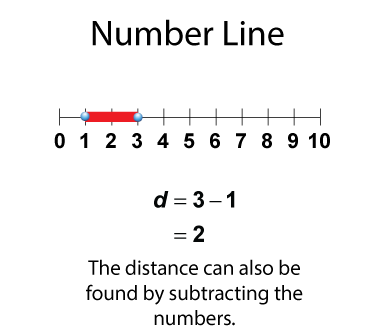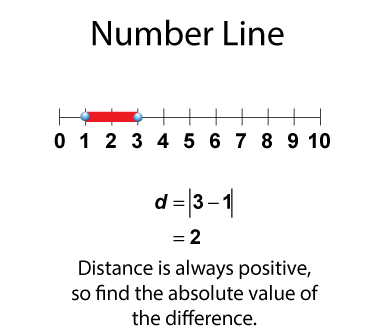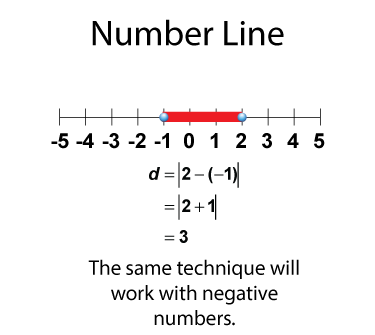# Worksheet: Number Line Subtraction

## Distance Measures on a Number Line

### A Brief Review of Number Lines

A number line is a horizontal line with equally spaced tic marks representing numbers.You can use a number line to represent numbers on a number line.### Distance on a Number Line

For any two numbers on a number line, you can find the distance between the two numbers.You can find the distance between the two numbers by subtracting.Distance is always positive, so find the absolute value of the difference.This technique will also work with negative numbers.Students use a number line to subtract numbers.